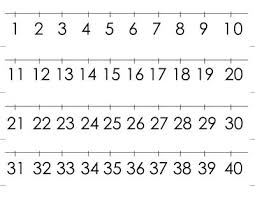# Positive 70974

The sum of two positive numbers is 2.5. A smaller number is four times smaller than a larger number. What is the value of a smaller number?

b =  0.5

### Step-by-step explanation:Did you find an error or inaccuracy? Feel free to write us. Thank you!

Tips for related online calculators
Do you have a linear equation or system of equations and looking for its solution? Or do you have a quadratic equation?